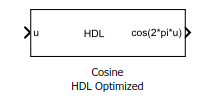# Sine HDL Optimized and Cosine HDL Optimized

Implement fixed-point sine and cosine wave by using lookup table approach optimized for HDL code generation

• Library:
• HDL Coder / Lookup Tables

••## Description

The Sine HDL Optimized block and Cosine HDL Optimized block implements a fixed-point sine or cosine wave by using a lookup table method that exploits quarter-wave symmetry.

For the most efficient HDL implementation, configure the block with an exact power of two as the number of elements. In the Block Parameters dialog box, for Number of data points, specify an integer that is an exact power of two. That is, specify the lookup table data points to be `(2^n)`, where n is an integer. By default, the Number of data points is 64.

When you specify a power of two for the Number of data points, the lookup tables precede a register without reset after HDL code generation. The combination of the lookup table block and register without reset maps efficiently to RAM on the target device.

Depending on your selection of the Output formula parameter, the blocks can output these functions of the input signal:

• sin(2πu)

• cos(2πu)

• exp(iu)

• sin(2πu) and cos(2πu)

Use the Table data type parameter to specify the word length of the fixed-point output data type. The fraction length of the output is the output word length minus 2.

## Ports

### Input

expand all

Input signal, u, specified as a real-valued scalar, vector, or matrix.

Data Types: `single` | `double` | `int8` | `int16` | `int32` | `int64` | `uint8` | `uint16` | `uint32` | `uint64` | `Boolean` | `fixed point` | `enumerated` | `bus`

### Output

expand all

Fixed-point sine wave, implemented using a lookup table approach.

#### Dependencies

This port is enabled when the Output formula is set to `sin(2*pi*u)` or ```sin(2*pi*u) and cos(2*pi*u)```.

Data Types: `fixed point`

Fixed-point cosine wave, implemented using a lookup table approach.

#### Dependencies

This port is enabled when the Output formula is set to `cos(2*pi*u)` or ```sin(2*pi*u) and cos(2*pi*u)```.

Data Types: `fixed point`

Complex exponential, implemented using a lookup table approach.

#### Dependencies

This port is enabled when the Output formula is set to `exp(j*2*pi*u)`.

Data Types: `fixed point`

## Parameters

expand all

Select the signal(s) to output.

Specify the number of data points to retrieve from the lookup table. The implementation is most efficient when you specify the lookup table data points to be `(2^n)`, where n is an integer.

Specify the table data type. You can specify an expression that evaluates to a data type, for example, `fixdt(1,16,0)`.

Click the buttonto display the Data Type Assistant, which helps you set the table data type.

Select the mode of data type specification. If you select `Expression`, enter an expression that evaluates to a data type, for example, `fixdt(1,16,0)`.

If you select `Fixed point`, you can use the options in the Data Type Assistant to specify the fixed-point data type. In the `Fixed point` mode, you can choose binary point scaling, and specify the signedness, word length, fraction length, and the data type override setting.

Selecting this check box inserts a unit delay without reset. You can simulate this delay in the Simulink® modeling environment. When you generate HDL code, a no-reset register is inserted after the block. The combination of lookup table with no-reset register maps to RAM on the target hardware.

## Version History

Introduced in R2016b